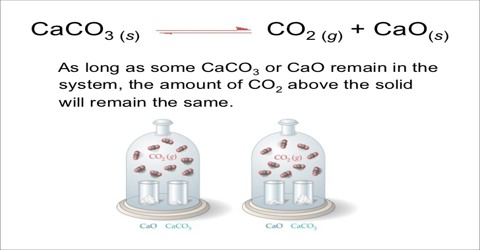Chemistry

# Heterogeneous EquilibriaHeterogeneous Equilibria

A homogeneous equilibrium occurs when all reagents and products are found in the same phase (solid, liquid, or gas) and a heterogeneous equilibrium is when they are in different phases. The position of heterogeneous equilibrium does not depend on the amount of pure solid and liquid present.

Consider the decomposition of calcium carbonate when heated to a high temperature:

CaCO3 (s) ↔ CaO (s) + CO2 (s)

There are three phases at equilibrium. The equilibrium constant in terms of partial pressures may be written as;

KP = PCO2

since at any temperature PCaO and PCaCO3 are considered to be constant. The value of the equilibrium constant at any temperature is determined solely by the equilibrium pressure of carbon dioxide evolved. This has been proved by experiments.If, however, two or more gaseous substances are involved in the reaction the situation becomes complicated. For example, for the dissociation of solid ammonium hydrogen sulphide according to the equation

NH4HS (s) ↔ NH3 (g) + H2S (g)

the equilibrium constant, KP, is

KP = PNH3 x PH2S

Here the equilibrium constant is the product of the partial pressure of ammonia and hydrogen sulphide gas. It the partial pressure of one of the two is increased, the partial pressure of the other must decrease so that KP remains the same.

Evaporation of a liquid and sublimation of a solid may also be regarded as examples of heterogeneous equilibria in so far as more than one phase arc existing together at equilibrium. Hence the vapour pressure of a liquid or a solid is one type of equilibrium constant. But these are physical equilibria.

Another type of physical equilibrium is a saturated solution in contact with the solute

Solid solute + aq ↔ Saturated solution

The equilibrium constant in its most correct form is-

K’ = asolution/asolute

where a stands for activity. The solute being solid its activity is taken as unity so that K’ = asolution. As the solution is saturated at the temperature may be replaced by solubility, S, and we obtain;

K = Solubility (S)